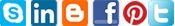Calculation of Maximum Internal Pressure of Steel Pipe
Date:2019-05-16      View(s):161      Tag:Maximum Pressure of Steel Pipe
Formula: P=2T[S]/D
P——The internal pressure of seamless pipe, MPa. D——The pipe inner diameter, mm. [S]——The allowable tensile stress strength of the pipe, MPa. T——Pipe wall thickness, mm.

This formula is obtained by taking a force analysis of a water pipe of unit length.

This means taking a water pipe of unit length and cutting it, taking out half, and studying the balance between the water pressure acting on it and the tensile force of the pipe wall to obtain this formula.

(The water pressure is PD, the wall tension is 2T[S], the two are equal, that is, PD=2T[S], and the two sides are divided by D to get the formula.)

The maximum withstand pressure is the pressure that can be withstood when the safety factor is equal to one.

The above formula does not consider the safety factor. If the safety factor K (K is greater than 1) is considered, then P = 2T[S] / (KD).

This formula can be used for seamless pipes, welded pipes and stainless steel pipes, but the allowable tensile stress [S] of the pipes in the formula is different, and the [S] of the welded pipe depends on the quality of the weld.

It should be emphasized that the pressure here refers to the internal water pressure of the pipeline, not the pressure outside the pipeline.
Products Category